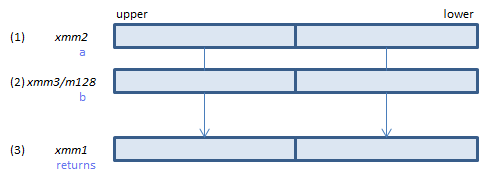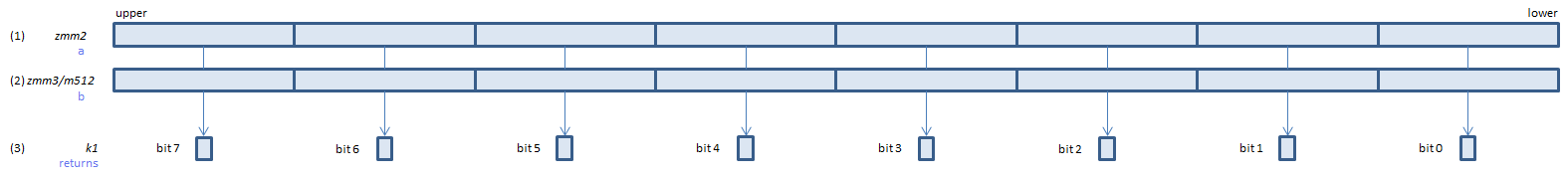﻿ vcmpgepd

## VCMPGEPD - CoMPare Greater Equal Packed Double

VCMPGEPD xmm1, xmm2, xmm3/m128    (V1
__m128d _mm_cmpge_pd(__m128d a, __m128d b)    (S2For each double, if (1) >= (2) set 1, else set 0, to all bits of the corresponding double of (3).
VCMPGEPD ymm1, ymm2, ymm3/m256    (V1
__m256d _mm256_cmp_pd(__m256d a, __m256d b, _CMP_GE_OS)For each double, if (1) >= (2) set 1, else set 0, to all bits of the corresponding double of (3).
VCMPGEPD k1{k2}, xmm2, xmm3/m128/m64bcst    (V5+VLFor each double, if (1) >= (2) set 1, else set 0, to the corresponding bit of (3).
If k2 bit is 0, the comparison is not done and the corresponding bit of (3) is set to zero. Upper bits of (3) are zero cleared.
VCMPGEPD k1{k2}, ymm2, ymm3/m256/m64bcst    (V5+VLFor each double, if (1) >= (2) set 1, else set 0, to the corresponding bit of (3).
If k2 bit is 0, the comparison is not done and the corresponding bit of (3) is set to zero. Upper bits of (3) are zero cleared.
VCMPGEPD k1{k2}, zmm2, zmm3/m512/m64bcst{sae}    (V5